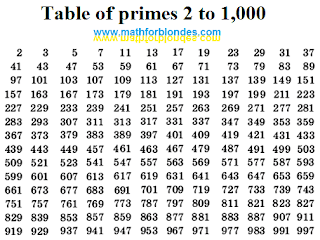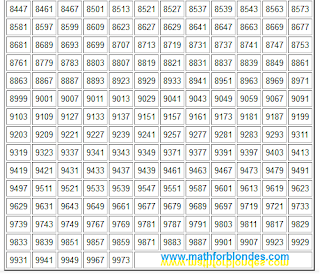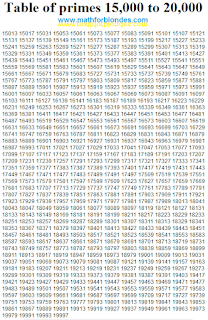## 10/17/2023

### Table of primes

A prime number (or a prime) is a natural number greater than 1 that is not a product of two smaller natural numbers. Prime numbers have exactly two divisors: 1 and the number itself. Below is a table of prime numbers up to 20000.Table of primes 2 to 1,000Table of primes 1,000 to 10,000Table of primes 10,000 to 15,000Table of primes 15,000 to 20,000

Prime numbers are a favorite toy of mathematicians. As of January 2019, the largest prime number known to mathematicians had 24,862,048 decimal digits. This prime numbers game is so addicting that even I am addicted to this game. What can I say about this? Playing prime numbers is very time-consuming and tedious, but the results can be very interesting. I came up with my own rules of the game, which I will tell you about soon. I hope this will be of interest to mathematicians.

I needed prime numbers up to 20,000. Here you will find prime numbers up to 21,000,000.